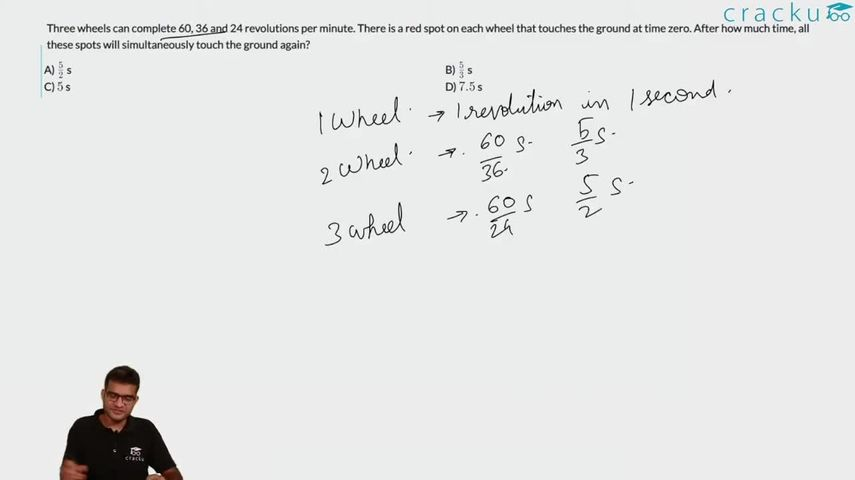Question 61

# Three wheels can complete 60, 36 and 24 revolutions per minute. There is a red spot on each wheel that touches the ground at time zero. After how much time, all these spots will simultaneously touch the ground again?

Solution

The first wheel completes a revolution in $$\frac{60}{60}=1$$ second
The second wheel completes a revolution in $$\frac{60}{36}=1\frac{2}{3}$$ second
The third wheel completes a revolution in $$\frac{60}{24}=2\frac{1}{2}$$ second

The three wheels touch the ground simultaneously at time which are multiples of the above three times.
Hence, the required number is $$LCM(1,\frac{5}{3},\frac{5}{2}) = 5$$ seconds.

So, the correct option is option (c)

### View Video Solution